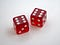Image by Brett Jordan available at Unsplash

# Understanding Probability Distribution

What is Probability Distribution? What are different types of Probability distributions? How will it help in framing Data Science Solutions?.

Let me try to explain it in very simple words

Definition:-A probability distribution is the mathematical function that gives the probabilities of occurrence of different possible outcomes for an experiment.

Rolling a Dice gives me a set of outcomes distributed in a particular way , where as the marks of a particular subject of a class gives me another distribution and occurrence of car accidents in a particular year follows an entirely different distribution and so on. Different Distributions help…

# Exploratory Data Analysis

When we hear about Data science or Analytics , the first thing that comes to our mind is Modelling , Tuning etc. . But one of the most important and primary steps before all of these is Exploratory Data Analysis or EDA.## Vivek Menon

An Enthusiastic Learner in the field of Artificial Intelligence, Machine Learning and Neural Networks. https://www.linkedin.com/in/viveksmenon/